Search

Cramer's RuleGiven a system of linear equations, Cramer's Rule is a handy way to solve for just one of the variables without having to solve the whole system of equations. They don't usually teach Cramer's Rule this way, but this is supposed to be the point of the Rule: instead of solving the entire system of equations, you can use Cramer's to solve for just one single variable.

Let's use the following system of equations:

2x +   y + z = 3

x    y  z = 0

x + 2y + z = 0

We have the left-hand side of the system with the variables (the "coefficient matrix") and the right-hand side with the answer values. Let D be the determinant of the coefficient matrix of the above system, and let Dx be the determinant formed by replacing the x-column values with the answer-column values:

 system ofequations coefficientmatrix'sdeterminant answercolumn Dx: coefficient determinantwith answer-columnvalues in x-column 2x + 1y + 1z = 3  1x  1y  1z = 0 1x + 2y + 1z = 0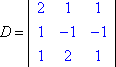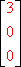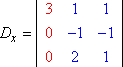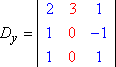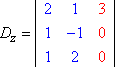Evaluating each determinant (using the method explained here), we get: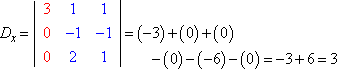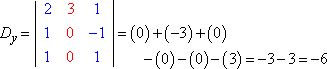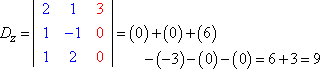Cramer's Rule says that x = Dx ÷ D, y = Dy ÷ D, and z = Dz ÷ D. That is:

x = 3/3 = 1,  y = 6/3 = 2,  and  z = 9/3 = 3

That's all there is to Cramer's Rule. To find whichever variable you want (call it "ß" or "beta"), just evaluate the determinant quotient Dß ÷ D. (Please don't ask me to explain why this works. Just trust me that determinants can work many kinds of magic.)

• Given the following system of equations, find the value of z.
• 2x +   y +   z = 1

x    y + 4z = 0

x + 2y  2z = 3

To solve only for z, I first find the coefficient determinant.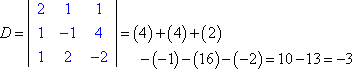Then I form Dz by replacing the third column of values with the answer column: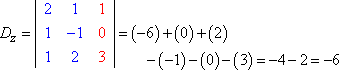Then I form the quotient and simplify: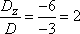z = 2

The point of Cramer's Rule is that you don't have to solve the whole system to get the one value you need. This saved me a fair amount of time on some physics tests. I forget what we were working on (something with wires and currents, I think), but Cramer's Rule was so much faster than any other solution method (and God knows I needed the extra time). Don't let all the subscripts and stuff confuse you; the Rule is really pretty simple. You just pick the variable you want to solve for, replace that variable's column of values in the coefficient determinant with the answer-column's values, evaluate that determinant, and divide by the coefficient determinant. That's all there is to it.

Almost.

What if the coefficient determinant is zero? You can't divide by zero, so what does this mean? I can't go into the technicalities here, but "D = 0" means that the system of equations has no unique solution. The system may be inconsistent (no solution at all) or dependent (an infinite solution, which may be expressed as a parametric solution such as "(a, a + 3, a  4)"). In terms of Cramer's Rule, "D = 0" means that you'll have to use some other method (such as matrix row operations) to solve the system. If D = 0, you can't use Cramer's Rule.